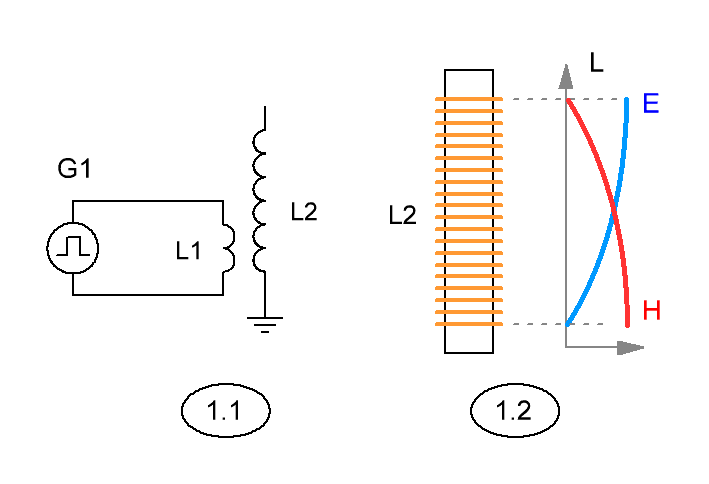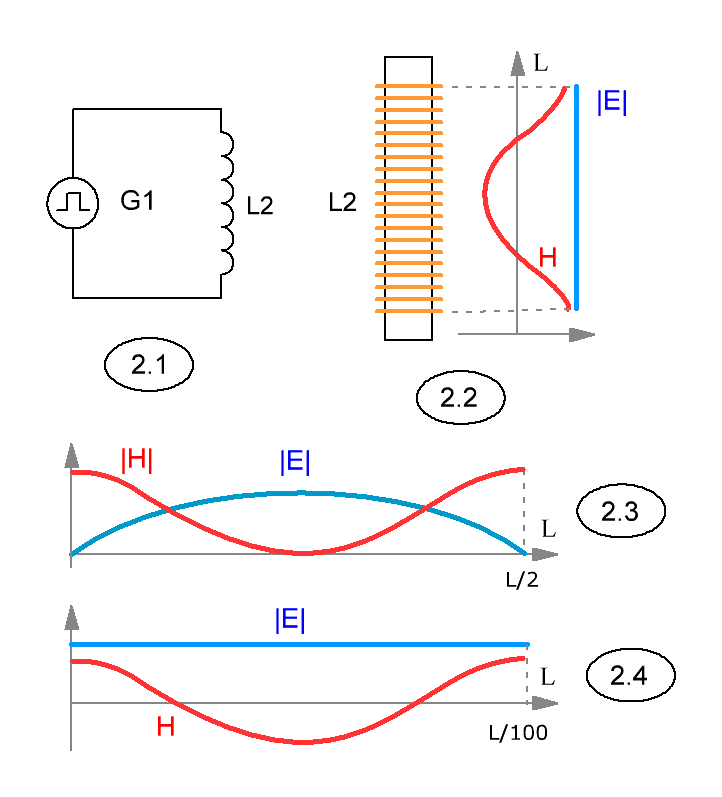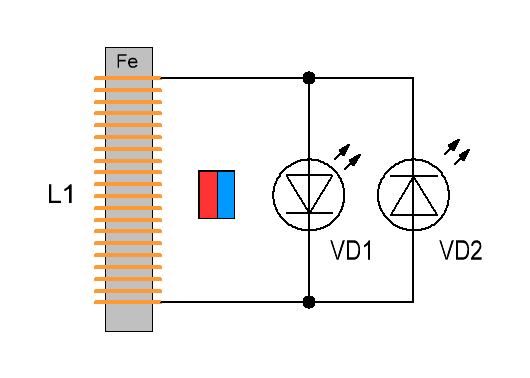Research website of Vyacheslav Gorchilin
2018-09-24
Slow magnetic waves
For many researchers and lovers of physics, this data can become a revelation, because sometimes partially, and sometimes completely they will go beyond classical concepts of magnetic field and wave processes in the long line. In this post we will talk about experiments and equipment for them, discuss their unusual results, and then talk about the generator for this effect.
Of course we know about radio wave processes occurring in long lines (DL). They are well-described by the Telegraph equations and the theory of Maxwell, are often used in practice and studied experimentally. The main difference from a long line concentrated is the distribution of loops of voltage and current along it. FOR such distribution begins, provided its length is equal to 1/4 of the wavelength, and the wave propagates along the DL with about the speed of light. In concentrated lines, loops of current and voltage is not observed, because the length of such lines is much less than the wavelength. The way it was before the opening of the slow waves, which later D. Smith called magnetic.Consider a simple circuit with generator G1, connected to a long line L2 through the inductor L1 (Fig. 1.1). By the way, is the classic scheme of the Tesla transformer. We find that the frequency of the oscillator so that the length DL equal to 1/4 of its wavelength. In this case, set the mode with the antinode of the current at the beginning of the DL and a voltage antinode at its end that is fully consistent with the classical ideas. For a magnetic field, this means its maximum at the beginning of the DL, and the maximum electric — in its end, as shown in figure (1.2).
Do you think that in reality it is the distribution of the magnetic field (MP) along the DL, if we start to reduce the frequency of the generator? From the standpoint of classical radio we get the scheme with lumped parameters, where shall be no redistribution of current and voltage. But is it really? Gradually reducing the frequency of the oscillator first, we will get to the 1/8 mode wavelength, where, under certain conditions, we may see the first non-classical phenomenon — the lack of MP around the conductor through which the current flows. In order to observe an even more unusual behavior MP, we will need to go down the generator to a frequency corresponding to 1/100 (or less) of the wavelength L2. It would seem, here where there is no redistribution of MP we can't see, but that's where the fun begins!
Non-classical distribution of the magnetic field
For the study of the unusual, from the point of view of the classics, the behavior of MP will collect even more simple scheme (Fig. 2.2). By the way, it is possible to leave the previous (1.1), but since here the electric field here doesn't reveal any anomalies, and the free end of the L2 is not needed, and the inductor L1 from the circuit can be removed.Those of our readers who have never turned their attention to this anomaly, will be very surprised, because instead of the expected continuous distribution of MP along the length of the coil, in reality we will see a very different picture.
The distribution of MP in the coil will be as shown in (2.2), where positive values of H correspond to one pole and negative to the other. This corresponds to the full wave distribution in classical DL with two obvious differences: the electric field (E) here continuously over the entire length, and the graph of the distribution of magnetic offset and is shown without the module! These differences are shown in figures 2.3 (the distribution of magnetic and electric fields in a classic full wave DL) and 2.4 (the distribution of magnetic and electric fields with slow waves).
But how is this possible? Indeed, the frequency of the generator G1 corresponds to hundredths classic wavelength. Here we must recall that in the theory of long lines, wave propagation speed near light, and from it we reckon the wavelength. To be more precise, the classical wave speed in DL is considered as the speed of light multiplied by a factor that takes into account the properties of the conductor. But for copper, or even wire insulation it will value slightly different from unity, but not of the order of 1/100. So, we are dealing with a fundamentally different type of waves! Next, we will continue our study of the unusual properties of these fields and the waves we call slow or magnetic.

Example. Coil L2 is wound wire of 2mm diameter plastic frame with a diameter of 50mm. The number of turns is 150. The wire length of 23.5 m. the Frequency generator G1 to receive the 1/4 wave in this DL needs to be 3.2 MHz. But for slow waves the frequency of the generator will be of the order of 15kHz

By the way, at least the electric field and is shown in figure (2.4), actually represents a very small value, which makes sense for such low frequencies.
The magnetic field indicator
To obtain a more objective picture of distribution of MP with a slow wave of one of the compass will not be enough. Not suitable and testers measure the magnitude of MP.Fig.3. The MP indicator showing the direction of its lines of force
The fact that all of these devices can not determine the direction of the magnetic lines of force in all planes. Oddly enough, but to help us in this can quite a simple indicator, which is presented on the left. To cut ferrite rod with a length of 3-5cm and a permeability of 400-600NM, wrap-copper wire diameter 0.3-0.4 mm in the amount of 50-70 turns. To the ends of the thus wound coil podpisatsja two counter is enabled LEDs VD1, VD2. LEDs themselves it is advisable to choose from the category of super-bright, the voltage of 1.5 V, then the sensitivity of the indicator will increase considerably. In subsequent figures, led this indicator conventionally, we will denote blue and red.
Still the best results for sensitivity will give the ferrite bead used in power filters or from a monitor cable. When selecting a ferrite should be guided by the following principle: it must be two distinct poles, and it should not be too high.
To use this indicator is also quite simple: you will need to carry it along the investigated coil, and, as the coaxial and perpendicular to the plane. About these studies and about the generator for slow waves, read the next section.